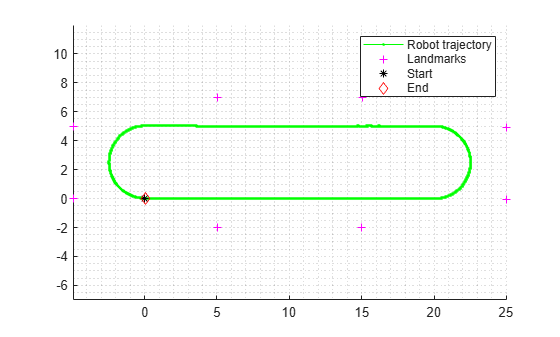# predict

Predict state and state error covariance

## Syntax

``predict(slamObj,controlInput)``
``predict(slamObj,controlInput,varargin)``

## Description

````predict(slamObj,controlInput)` predicts the state and state error covariance. `predict` uses the StateTransitionFcn property of the `ekfSLAM` object, `slamObj`, and the controller input `controlInput` to predict the state.```

example

````predict(slamObj,controlInput,varargin)` passes all additional arguments specified in `varargin` to the underlying StateTransitionFcn property of `slamObj`.The first input to `StateTransitionFcn` is the pose from the previous time step, followed by all user-defined arguments in `varargin`.```

## Examples

collapse all

Load a race track data set that contains the initial vehicle state, initial vehicle state covariance, process noise covariance, control input, time step size, measurement, measurement covariance, and validation gate values.

```load("racetrackDataset.mat","initialState","initialStateCovariance", ... "processNoise","controllerInputs","timeStep", ... "measurements","measCovar","validationGate");```

Create an `ekfSLAM` object with initial state, initial state covariance, and process noise.

```ekfSlamObj = ekfSLAM("State",initialState, ... "StateCovariance",initialStateCovariance, ... "ProcessNoise",processNoise);```

Initialize a variable to store the pose.

```storedPose = nan(size(controllerInputs,1)+1,3); storedPose(1,:) = ekfSlamObj.State(1:3);```

Predict the state using the control input and time step size for the state transition function. Then, correct the state using the data of the observed landmarks, measurement covariance, and validation gate for the data association function.

```for count = 1:size(controllerInputs,1) % Predict the state predict(ekfSlamObj,controllerInputs(count,:),timeStep); % Get the landmarks in the environment observedLandmarks = measurements{count}; % Correct the state if ~isempty(observedLandmarks) correct(ekfSlamObj,observedLandmarks, ... measCovar,validationGate); end % Log the estimated pose storedPose(count+1,:) = ekfSlamObj.State(1:3); end```

Visualize the created map.

```fig = figure; figAx = axes(fig); axis equal grid minor hold on plot(figAx,storedPose(:,1),storedPose(:,2),"g.-") landmarks = reshape(ekfSlamObj.State(4:end),2,[])'; plot(figAx,landmarks(:,1),landmarks(:,2),"m+") plot(figAx,storedPose(1,1),storedPose(1,2),"k*") plot(figAx,storedPose(end,1),storedPose(end,2),"rd") legend("Robot trajectory","Landmarks","Start","End")```## Input Arguments

collapse all

EKF SLAM object, specified as a `ekfSLAM` object.

Controller input required to propagate the state from initial value to final value, specified as an N-element vector.

Note

The dimension of the process noise must be equal to the number of elements in `controlInput`.

Data Types: `single` | `double`

Variable-length input argument list, specified as a comma-separated list. This input is passed directly into the StateTransitionFcn property of `slamObj` to evolve the state. When you call:

`predict(slamObj,controlInput,arg1,arg2)`

MATLAB® essentially calls the `stateTransitionFcn` as:

`stateTransitionFcn(pose(k-1),controlInput,arg1,arg2)`

## Version History

Introduced in R2021b